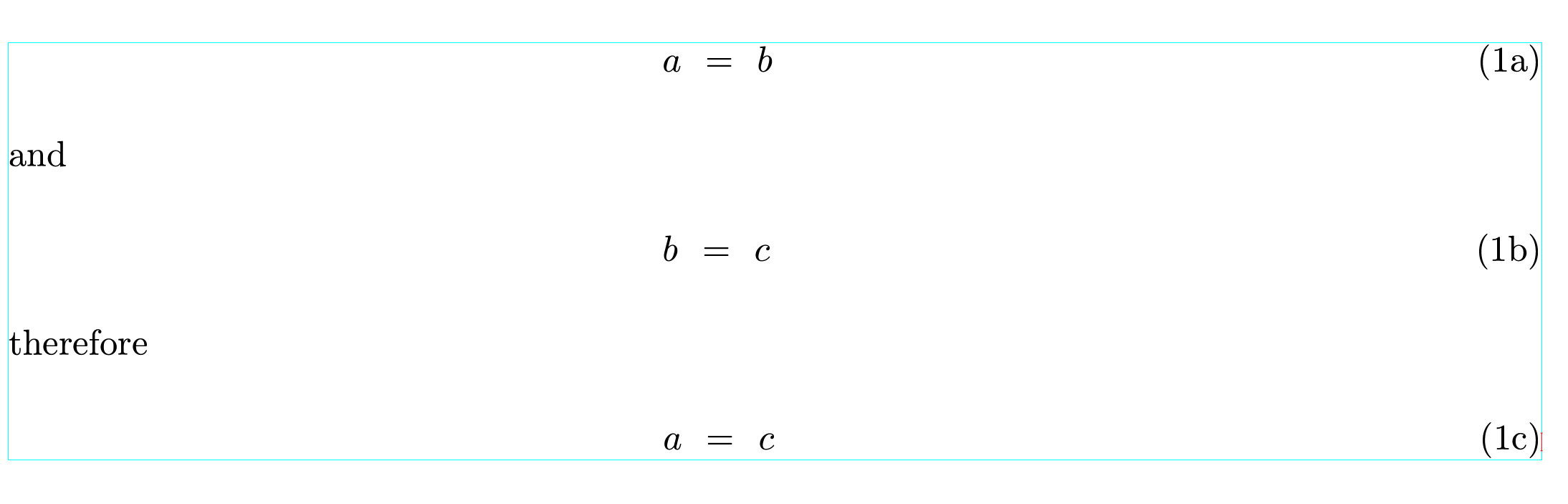# Numeration of subequation like 1a, 1b etc

In the amsmath package of Latex, there is environment subequations which allow numerate subequations as 1a, 1b etc. How it may be realized in TeXMacs?

A first quick, imperfect attempt is the following:

<assign|subequation|<macro|body|<next-equation><new-counter|subequation><with|next-number|<macro|<next-subequation><with|mode|text|font-shape|right|(<the-equation><number|<the-subequation>|alpha>)>>|<arg|body>>>>


This lets you put a subequation tag around a “Several equations” tag making a subcounter for those equations. It doesn’t work with a “Displayed formula”.

Thank you. It works, but the number of equations is out of text width. Another problem.
The converted to Latex file can not be compiled. In preamble appears new command
\newcommand{\subequation}{{\nextequation}{\newcounter{subequation}}#1} and the equations with this operator is
{\subequation{\begin{eqnarray}
bbb&=&nnnn\
nnnn&=& mmmm
\end{eqnarray}}}.
Error during the compilation is Undefined control sequence. \end{eqnarray}}

After some re-reading of Section 12.4.3 of The Jolly Writer, here is a second attempt, which deals with “Displayed formulae” as well.

<assign|subequation|<\macro|body>
<surround|<next-equation><new-counter|subequation>||<with|equation|<\macro|body>
<\surround|<next-subequation>|>
<\equation-lab>
<arg|body>
</equation-lab|<the-equation><number|<the-subequation>|alpha>>
</surround>
</macro>|next-number|<macro|<next-subequation><with|mode|text|font-shape|right|(<the-equation><number|<the-subequation>|alpha>)>>|<arg|body>>>
</macro>>


As for LaTeX conversion, the same comments would apply as they did to your previous question: identical macros in TeXmacs and LaTeX are probably the way to go. I haven’t had time to experiment with that yet. I personally don’t make use of that functionality.

Very nice macro.

If anyone else has experience with this (in particular, the developers), it would be a very nice feature to be able to customize the LaTeX export; in this way one could both define a new macro (as @jeroen did) and specify how it should be exported to LaTeX (important for people that want to export to LaTeX)

I also both unselected “Allow for macro definitions in the preamble” and selected “Expand user-defined macros” but I did not get a compilable TeXmacs document.

Thank you very much. It works in TexMacs with the right position of the numbers of equations. Unfortunately, I can not produce the code you made. It is a bad side of TeXMacs - to write something I have to reads first Jolly Book. Because this sub-numbering is sometimes needed in scientific papers, it will be better to include this possibility into Texnacs code.

It does not work in converted to Latex file. I understand that you have no time for more experiments, but I put below what happened in the Latex file. Maybe somebody can correct it. In preamble appears macros

\newcommand{\subequation}{{\nextequation}{\newcounter{subequation}}#1}

And the application in an equation is

{\subequation{\begin{eqnarray}
aaa & = & bbb, \
ccc & = ddd.
\end{eqnarray}}}

with the same error as before Undefined control sequence. \end{eqnarray}}

Simple solution for me is change macros in Latex file to
\newcommand{\subequation}{\begin{subequations} #1\end{subequations}}
It works well. But Latex->Texmacs does not work for this definition because TexMacs does not know environment subequation.

Below I wrote simple solution - redefine macros in Latex file to
\newcommand{\subequation}{\begin{subequations} #1\end{subequations}}
and naturally, you need package amsmath for environment subequation. But this solution does not work in the Latex -> Texmacs convert. The macros made of @jeroen is working for a separate group of the equation like eqnarry, but invirornment subequation from amsmath works for a group of different collection of equation and text. You can put part of the paper inside of this environment, a combination of text and equations.

I support your suggestion to include this possibility in Texmacs code.

I made some progress using Format->Specific->Latex (see http://www.texmacs.org/tmweb/manual/webman-convert.en.html) to have the LaTeX macro you wrote exported into the LaTeX file, but not seen by TeXmacs. However, I can only export it into the main text, even if I wrote it in the preamble.

Maybe I shall ask the question on the mailing list (JvdH reads that more than the forum).

@JoePass I expect that over time much of the frequently-used LaTeX functionality will become available in the main TeXmacs distribution or as external packages. This would gradually reduce the need to understand macro definition or Scheme programming. Indeed, it appears there was already a placeholder for subequations in the TeXmacs code. I have submitted a pull request to implement it.

If I understand correctly what you mean, this should also work in TeXmacs:I think I made it work.
You need to

• Put the macro you defined in the TeXmacs preamble, inside a \specific|latex macro:
<specific|latex|\newcommand{\subequation}{\begin{subequations}#1\end{subequations}}>
• From Edit->Preferences->Converters->(TeXmacs->Latex) deselect “Expand unrecognized macros”
—but here I am not confident that I recall how I changed the options, as I tried in different ways: they look like this

• You can also add in the preamble <specific|latex|\usepackage{amsmath}> (I think it should be before the macro)

Probably there isn’t the possibility of selecting the option of expanding or not expanding macro by macro. Maybe I will ask this in the mailing list rather than “how to” in general.

Yes, I was rong. It works for part of the text with formulas. Please explain to me how to apply this macro for already written text with formulas. If mark out text and then print the macro \subequation, then the text is already written disappeared.

Thank you, it works but with a single correction: change \newcommand to \renewcommand. The point is that in the converted file both definitions appeared. The first one is by @jeroen and the second one due to \specific. In Latex, the macros with the same name can not be together.

Try this small modification of Jeroen’s macro (I think it was already in my TeXmacs file when I wrote the post, but I forgot about it): wrap it inside a \specific|texmacs macro.
Now it will work for TeXmacs, but it will not be exported to LaTeX and you will have only one definition of \subequation

<specific|texmacs|<assign|subequation|<\macro|body>
<surround|<new-counter|subequation>||<with|equation|<\macro|body>
<\surround||>
<\equation-lab>
<arg|body>
</equation-lab|<number||alpha>>

|next-number|<macro|<with|mode|text|font-shape|right|(<number||alpha>)>>|<arg|body>>>
>>

Excellent. It works now with \newcommand and texmacs definition has no appeared in Latex file.